# Non-parametric Bayes: Gaussian Processes¶

Use of the term "non-parametric" in the context of Bayesian analysis is something of a misnomer. This is because the first and fundamental step in Bayesian modeling is to specify a full probability model for the problem at hand. It is rather difficult to explicitly state a full probability model without the use of probability functions, which are parametric. Bayesian non-parametric methods do not imply that there are no parameters, but rather that the number of parameters grows with the size of the dataset. In fact, Bayesian non-parametric models are infinitely parametric.

## Building models with Gaussians¶

It is easy to develop large, parametric models $p(y|\theta)$ for large $\theta$, particularly in a Bayesian context. However, this usually results in having to work with multidimensional integration over $\theta$.

One approach is to use MCMC; another is to represent your model with Gaussians. Normal distributions are easier to work with.

$$p(x \mid \pi, \Sigma) = (2\pi)^{-k/2}|\Sigma|^{-1/2} \exp\left\{ -\frac{1}{2} (x-\mu)^{\prime}\Sigma^{-1}(x-\mu) \right\}$$
• marginals of multivariate normal distributions are normal
$$p(x,y) = \mathcal{N}\left(\left[{ \begin{array}{c} {\mu_x} \\ {\mu_y} \\ \end{array} }\right], \left[{ \begin{array}{c} {\Sigma_x} & {\Sigma_{xy}} \\ {\Sigma_{xy}^T} & {\Sigma_y} \\ \end{array} }\right]\right)$$$$p(x) = \int p(x,y) dy = \mathcal{N}(\mu_x, \Sigma_x)$$
• conditionals of multivariate normals are normal
$$p(x|y) = \mathcal{N}(\mu_x + \Sigma_{xy}\Sigma_y^{-1}(y-\mu_y), \Sigma_x-\Sigma_{xy}\Sigma_y^{-1}\Sigma_{xy}^T)$$

In some situations, we want to gain inference about functions, rather than about, say, individuals or small vectors of parameters.

A Gaussian process generalizes the multivariate normal to infinite dimension. It is considered a non-parametric approach, despite having an infinite number of parameters.

Gaussian Process

An infinite collection of random variables, any finite subset of which have a Gaussian distribution.

A Gaussian process is a disribution over functions. Just as a multivariate normal distribution is completely specified by a mean vector and covariance matrix, a GP is fully specified by a mean function and a covariance function:

$$p(x) \sim \mathcal{GP}(m(x), k(x,x^{\prime}))$$

It is the marginalization property that makes working with a Gaussian process feasible: we can marginalize over the infinitely-many variables that we are not interested in, or have not observed.

For example, one specification of a GP might be as follows:

\begin{aligned} m(x) &=0 \\ k(x,x^{\prime}) &= \theta_1\exp\left(-\frac{\theta_2}{2}(x-x^{\prime})^2\right) \end{aligned}

here, the covariance function is a squared exponential, for which values of $x$ and $x^{\prime}$ that are close together result in values of $k$ closer to 1 and those that are far apart return values closer to zero. (spoiler: we usually aren't very interested in the mean function!).

For a finite number of points, the GP becomes a multivariate normal, with the mean and covariance as the mean functon and covariance function evaluated at those points.

In :
%matplotlib inline
import random
import numpy as np
import matplotlib.pyplot as plt
import seaborn as sns; sns.set_context('notebook')
random.seed(34738924)


We can generate a random sample from a GP with mean function zero and a double exponential covariance function as follows.

In the context of Gaussian Processes, the covariance matrix is referred to as the kernel (or Gram) matrix.

In :
# Covariance matrix for MV normal
covariance = lambda kernel, x, y, params: \
np.array([[kernel(xi, yi, params) for xi in x] for yi in y])


The exponential kernel:

In :
exponential_kernel = lambda x, y, params: params * \
np.exp( -0.5 * params * np.sum((x - y)**2) )

In :
x = np.random.randn(10)*2
theta = [1, 10]
sigma = covariance(exponential_kernel, x, x, theta)
y = np.random.multivariate_normal(np.zeros(len(x)), sigma)
plt.plot(x, y, 'bo');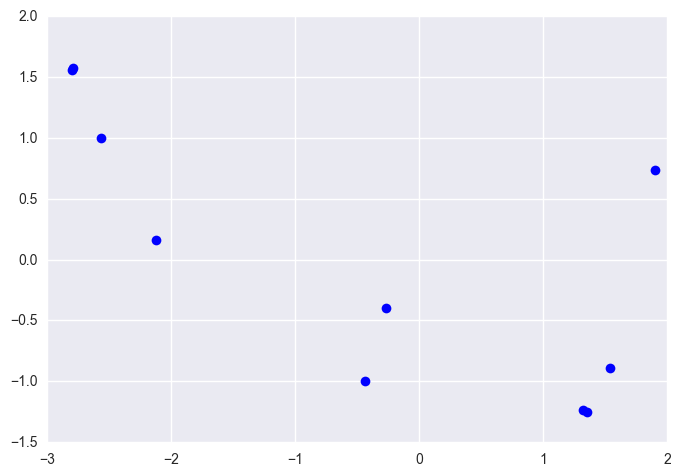### Conditional distribution¶

We can generate sample functions (realizations) sequentially, using the conditional:

$$p(x|y) = \mathcal{N}(\mu_x + \Sigma_{xy}\Sigma_y^{-1}(y-\mu_y), \Sigma_x-\Sigma_{xy}\Sigma_y^{-1}\Sigma_{xy}^T)$$

This function implements the conditional:

In :
def conditional(x_new, x, y, fcov=exponential_kernel, params=theta):
B = covariance(fcov, x_new, x, params)
C = covariance(fcov, x, x, params)
A = covariance(fcov, x_new, x_new, params)
mu = np.linalg.inv(C).dot(B).T.dot(y)
sigma = A - np.linalg.inv(C).dot(B).T.dot(B)
return(mu.squeeze(), sigma.squeeze())


The band plotted below represents the prior mean function, plus and minus one standard deviation.

In :
sigma0 = exponential_kernel(0, 0, theta)
xpts = np.arange(-3, 3, step=0.01)
plt.errorbar(xpts, np.zeros(len(xpts)), yerr=sigma0, capsize=0)
plt.ylim(-3, 3);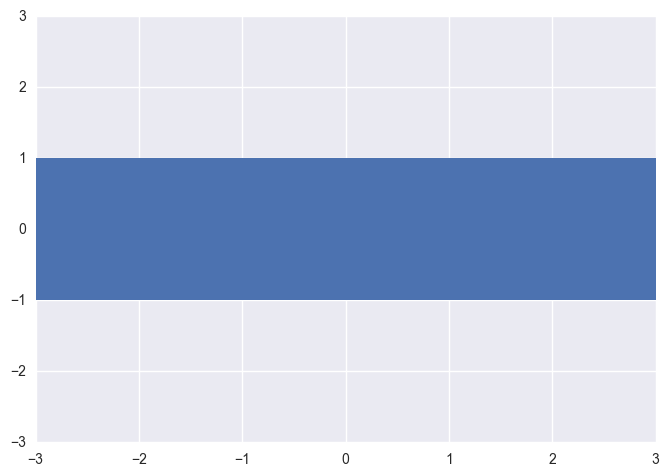We start by selecting a point at random, then drawing from an unconditional Gaussian:

In :
x = [1.]
y = [np.random.normal(scale=sigma0)]
y

Out:
[-1.2793668900024635]

We can now calculate the conditional distribution, given the point that we just sampled.

In :
sigma1 = covariance(exponential_kernel, x, x, theta)

In :
def predict(x, data, kernel, params, sigma, t):
k = [kernel(x, y, params) for y in data]
Sinv = np.linalg.inv(sigma)
y_pred = np.dot(k, Sinv).dot(t)
sigma_new = kernel(x, x, params) - np.dot(k, Sinv).dot(k)
return y_pred, sigma_new

In :
x_pred = np.linspace(-3, 3, 1000)
predictions = [predict(i, x, exponential_kernel, theta, sigma1, y)
for i in x_pred]


Let's plot this to get an idea of what this looks like:

In :
y_pred, sigmas = np.transpose(predictions)
plt.errorbar(x_pred, y_pred, yerr=sigmas, capsize=0)
plt.plot(x, y, "ro")
plt.xlim(-3, 3); plt.ylim(-3, 3);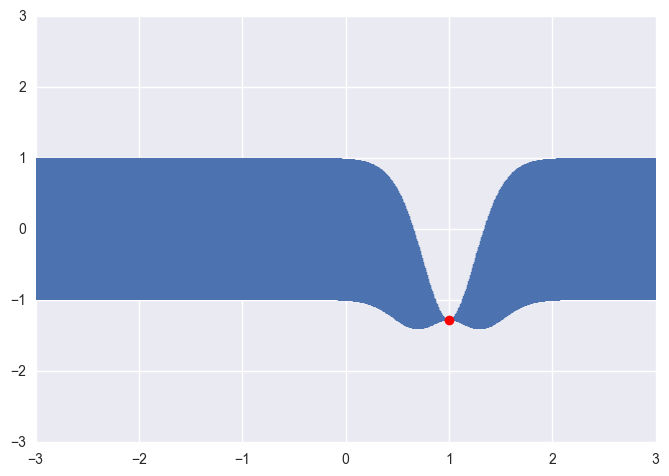Now, we can select a second point, conditional on the first point, using this new distribution. Let's arbitrarily select one at $x=-0.7$

In :
mu2, s2 = conditional([-0.7], x, y)
y2 = np.random.normal(mu2, s2)
y2

Out:
-0.3822530996980265
In :
x.append(-0.7)
y.append(y2)


We can calculate the conditional distribution again:

In :
sigma2 = covariance(exponential_kernel, x, x, theta)

In :
predictions = [predict(i, x, exponential_kernel, theta, sigma2, y)
for i in x_pred]

In :
y_pred, sigmas = np.transpose(predictions)
plt.errorbar(x_pred, y_pred, yerr=sigmas, capsize=0)
plt.plot(x, y, "ro")
plt.xlim(-3, 3); plt.ylim(-3, 3);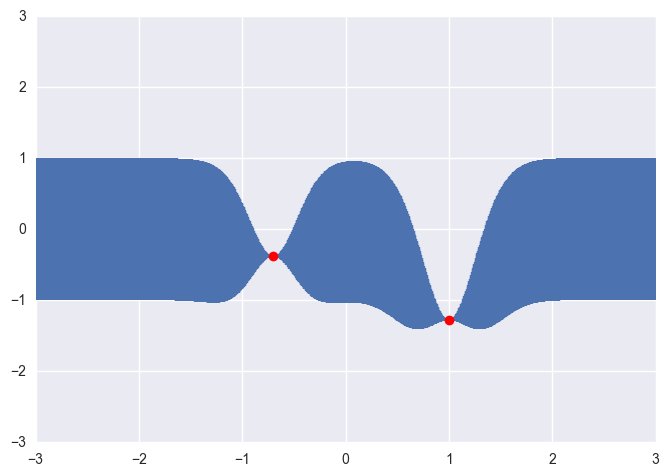Notice how the existing points constrain the selection of subsequent points.

In :
x_more = [-2.1, -1.5, 0.3, 1.8, 2.5]
mu, s = conditional(x_more, x, y)
y_more = np.random.multivariate_normal(mu, s)
y_more

Out:
array([ 0.24923286, -0.6789484 ,  0.0585416 ,  0.10131664,  0.59467425])
In :
x += x_more
y += y_more.tolist()

In :
sigma_new = covariance(exponential_kernel, x, x, theta)

predictions = [predict(i, x, exponential_kernel, theta, sigma_new, y)
for i in x_pred]

y_pred, sigmas = np.transpose(predictions)
plt.errorbar(x_pred, y_pred, yerr=sigmas, capsize=0)
plt.plot(x, y, "ro")
plt.ylim(-3, 3);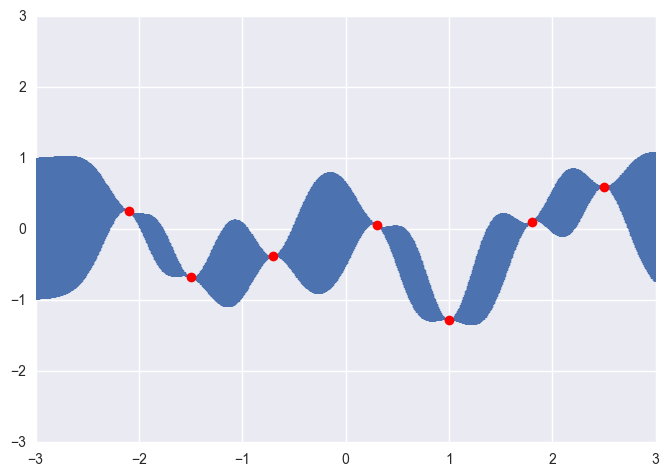Note that this is exactly equivalent to adding points simultaneously.

We are not restricted to a double-exponential covariance function. Here is a slightly more general function, which includes a constant and linear term, in addition to the exponential.

$$k(x,x\prime) = \theta_1 \exp\left(-\frac{\theta_2}{2}(x-x^{\prime})^2\right) + \theta_3 + \theta_4 x^{\prime} x$$
In :
# Exponential kernel, plus constant and linear terms
def exponential_linear_kernel(x, y, params):
return exponential_kernel(x, y, params[:2]) + params + params * np.dot(x, y)

In :
# Parameters for the expanded exponential kernel
theta = 2.0, 50.0, 0.0, 1.0

# Some sample training points.
xvals = np.random.rand(10) * 2 - 1

# Construct the Gram matrix
C = covariance(exponential_linear_kernel, xvals, xvals, theta)

# Sample from the multivariate normal
yvals = np.random.multivariate_normal(np.zeros(len(xvals)), C)

plt.plot(xvals, yvals, "ro");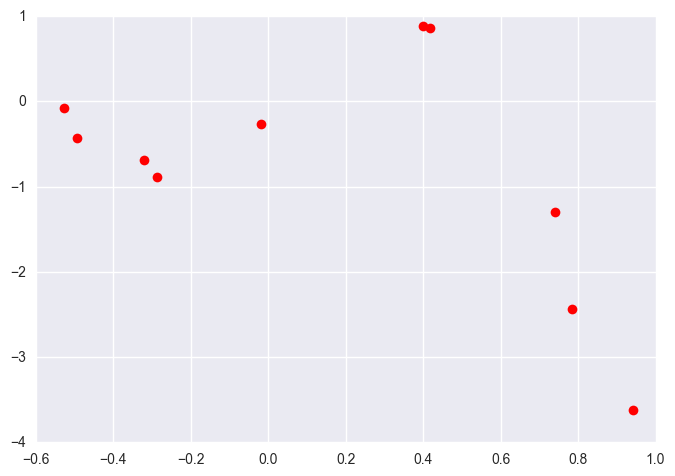In :
x_pred = np.linspace(-1, 1, 1000)
predictions = [predict(i, xvals, exponential_linear_kernel, theta, C, yvals)
for i in x_pred]

In :
y, sigma = np.transpose(predictions)
plt.errorbar(x_pred, y, yerr=sigma, capsize=0)
plt.plot(xvals, yvals, "ro");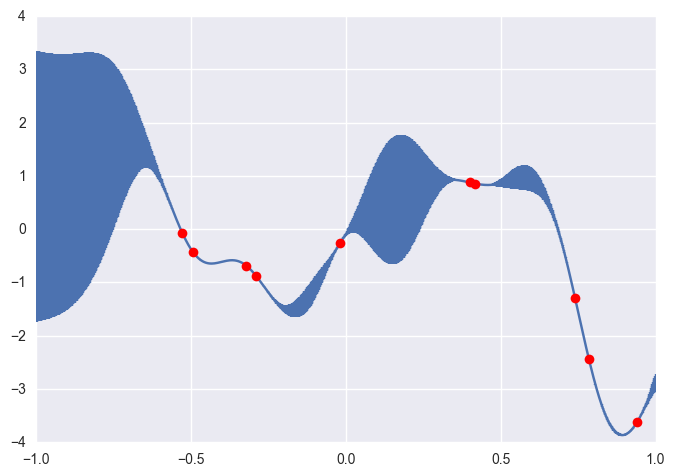We can use the GP as a prior, and update it using data (rather than random realizations), to obtain a posterior GP that we can use for prediction, conditional on the data.

## Marginal Likelihood¶

The marginal likelihood is the normalizing constant for the posterior distribution, and is the integral of the product of the likelihood and prior.

$$p(y|X) = \int_f p(y|f,X)p(f|X) df$$

where for Gaussian processes, we are marginalizing over function values $f$ (instead of parameters $\theta$).

GP prior:

$$\log p(f|X) = - \frac{k}{2}\log2\pi - \frac{1}{2}\log|K| -\frac{1}{2}f^TK^{-1}f$$

Gaussian likelihood:

$$\log p(y|f,X) = - \frac{k}{2}\log2\pi - \frac{1}{2}\log|\sigma^2I| -\frac{1}{2}(y-f)^T(\sigma^2I)^{-1}(y-f)$$

Marginal likelihood:

$$\log p(y|X) = - \frac{k}{2}\log2\pi - \frac{1}{2}\log|K + \sigma^2I| - \frac{1}{2}y^T(K+\sigma^2I)^{-1}y$$

Notice that the marginal likelihood includes both a data fit term $- \frac{1}{2}y^T(K+\sigma^2I)^{-1}y$ and a parameter penalty term $\frac{1}{2}\log|K + \sigma^2I|$. Hence, the marginal likelihood can help us select an appropriate covariance function, based on its fit to the dataset at hand.

### Choosing parameters¶

This is relevant because we have to make choices regarding the parameters of our Gaussian process; they were chosen arbitrarily for the random functions we demonstrated above.

For example, in the squared exponential covariance function, we must choose two parameters:

$$k(x,x^{\prime}) = \theta_1\exp\left(-\frac{\theta_2}{2}(x-x^{\prime})^2\right)$$

The first parameter $\theta_1$ is a scale parameter, which allows the function to yield values outside of the unit interval. The second parameter $\theta_2$ is a length scale parameter that determines the degree of covariance between $x$ and $x^{\prime}$; smaller values will tend to smooth the function relative to larger values.

We can use the marginal likelihood to select appropriate values for these parameters, since it trades off model fit with model complexity. Thus, an optimization procedure can be used to select values for $\theta$ that maximize the marginial likelihood.

These examples, of course, are trivial because they are simply random functions. What we are really interested in is learning about an underlying function from information residing in our data. In a parametric setting, we either specify a likelihood, which we then maximize with respect to the parameters, of a full probability model, for which we calculate the posterior in a Bayesian context. Though the integrals associated with posterior distributions are typically intractable for parametric models, they do not pose a problem with Gaussian processes.

## Gaussian process priors¶

We can treat our zero-mean (or otherwise arbitrary) Gaussian process as a prior for our model. If we are able to use a Gaussian as our data likelihood, then we can construct a Gaussian proceess posterior.

Keeping in mind that a Gaussian process is a distribution over functions, rather than parameters, our likelihood takes the following form:

$$y|p(x),x \sim \mathcal{N}(p(x), \sigma^2I)$$

Here, $\sigma^2$ represents observation error, or noise, and our unknown is a function.

Notice that the GP likelihood conditions on a function; however, we are only interested in the function at locations where we have data!

The corresponding prior is:

$$p(x) \sim \mathcal{GP}(m_0(x), k_0(x,x\prime))$$

Multiplying an infinite normal with another infinite normal yields another infinite normal, our posterior process:

$$p(x)|y \sim \mathcal{GP}(m_{post}, k_{post}(x,x^{\prime}))$$

where

\begin{aligned} m_{post} &= k(x,x^{\prime})^T[k(x,x) + \sigma^2I]^{-1}y \\ k_{post}(x,x^{\prime}) &= k(x^{\prime},x^{\prime}) - k(x,x^{\prime})^T[k(x,x) + \sigma^2I]^{-1}k(x,x^{\prime}) \end{aligned}

The posterior predictive distribution for the GP is specified by:

\begin{aligned} m^* &= k(x^*,x)^T[k(x,x) + \sigma^2I]^{-1}y \\ k^*(x^*,x) &= k(x^*,x^*)+\sigma^2 - k(x^*,x)^T[k(x,x) + \sigma^2I]^{-1}k(x^*,x) \end{aligned}

Though this calculation may seem straightforward, but note that the mean and covariate calculations involve inversions $k(x,x)$, which is a $\mathcal{O}(n^3)$ computation. Thus, Gaussian processes as presented are usually only feasible for data up to a few thousand observations in size. If time allows, we'll tak about sparse approximations.

## Gaussian Processes in GPy¶

Due to it's simplicity in syntax, we demonstrate several of the key components of GP regression using the package GPy. It is actively maintained by the Sheffield Computing Group.

In :
import GPy


For the purposes of demonstration, let's generate some toy data such that: \begin{equation} y_i = f(x_i)+\epsilon \end{equation}

where $f(x) = 400 + 30\sin(.2X)$ and $\epsilon\sim \mathcal{N}(0,15)$

In :
X = np.sort(10*np.random.randn(100)+10)
Y = 30*np.sin(.2*X)+np.random.multivariate_normal(np.repeat(0,100), 15*np.eye(100))
plt.plot(X, Y, "bo");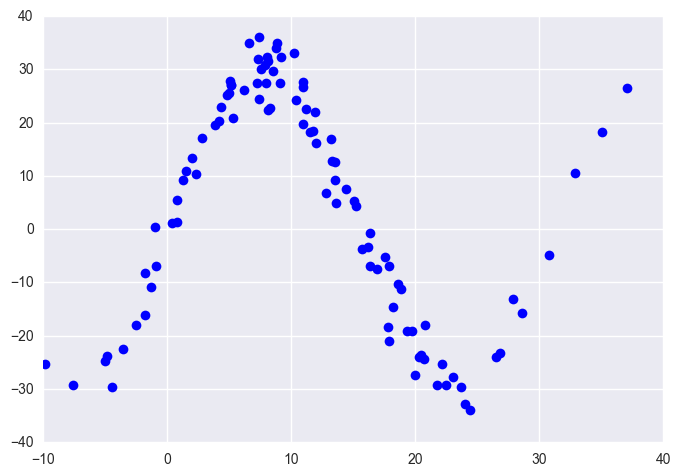Our goal is to use the GP infrastructure to try and recover the true function $f$.

### Mean function¶

The mean function of a GP can be interpreted as a "prior guess" at the form of the true function. Typically, we use a zero mean function (or some linear function), as we have seen above, but we can choose from a range of alternatives.

## Covariance function¶

The behavior of individual realizations from the GP is governed by the covariance function. This function controls both the degree of shrinkage to the mean function and the smoothness of functions sampled from the GP.

The Matèrn class of functions is a flexible choice.

$$k_{Matern}(d) = \frac{\sigma^2}{\Gamma(\nu)2^{\nu-1}} \left(\frac{\sqrt{2 \nu} d}{l}\right)^{\nu} K_{\nu}\left(\frac{\sqrt{2 \nu} d}{l}\right)$$

The Matèrn covariance function has three parameters, each of which clearly controls one of three important properties of realizations.

amplitude ($\sigma$) (sometimes called "variance" and parameterized by $\sigma^2$)

The amplitude parameter (amp in PyMC) is a multiplier for realizations from the function that essentially stretches or compresses the y-axis.

lengthscale of changes ($l$)

The lengthscale parameter (scale) similarly scales realizations on the x-axis. Larger (greater than 1) values make points appear closer together.

roughness ($\nu$)

The roughness parameter (diff_degree) controls the sharpness of the ridge of the covariance function, which in turn affects the roughness (or smoothness) of realizations.

• The kernel function relates inputs to each other. Intuition: input points that are closer together might have similar output values (of course, this depends...)
• Pairwise evaluations of the kernel function produces a positive semidefinite Gram matrix.

In GPy, specification of the kernel is very easy:

In :
kernel = GPy.kern.Matern32(1, variance=10, lengthscale=1.2)


Note, in GPy, the roughness is predetermined and comes in 2 separate Matern Functions

Calculation of the Gram matrix is very simple for our X:

In :
K_xx = kernel.K(X[:,None],X[:,None])
plt.imshow(np.asmatrix(K_xx), cmap='terrain', interpolation='nearest')
plt.axis('off');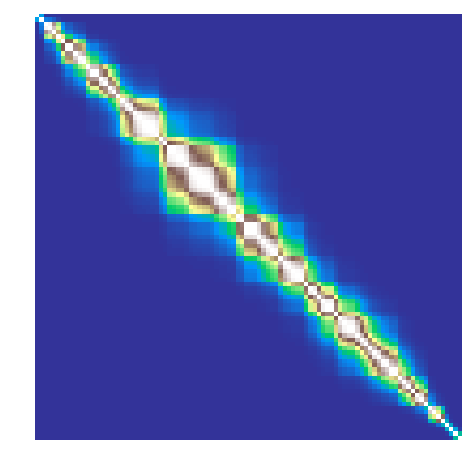### Exercise: hyperparameters¶

• Try playing around with the hyperparameters to see what we get.
• Can you hypothesize how this will affect the draws of the function?

## Drawing realizations from a GP¶

Since a Gaussian process is a distribution over functions, sampling from it yields functions rather than points. In practice, however, we can only observe a finite number of points on the function. Since, by definition, a GP is an infinite collection of random variables, any collection of which is multivariate Gaussian, then a draw from the prior process is practically speaking a draw from a multivariate Gaussian with Covariance equal to the Gram Matrix above.

In :
import scipy as sp
rv = sp.random.multivariate_normal(size = 2, mean = np.repeat(0,len(X)), cov = np.asmatrix(K_xx))
for draw in rv:
plt.plot(X, draw, "b-", alpha=.9);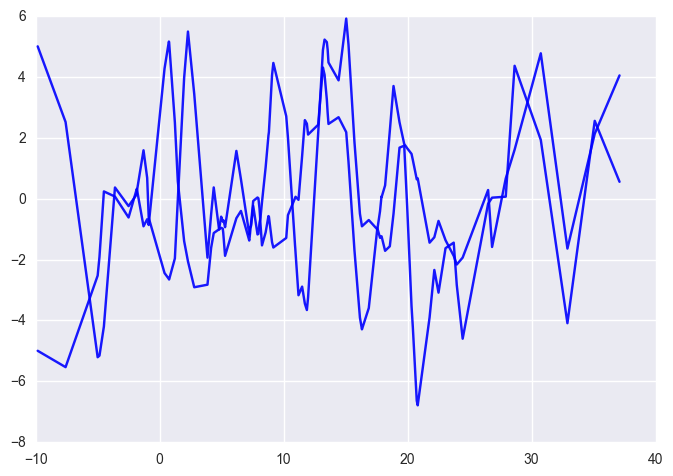### Exercise: hyperparameters¶

Try manipulating the hyperparameters. Does this match what you hypothesized?

Let's use a GP to model observations as coming from the toy function for which we are highly uncertain. We can model this data as:

$$\text{data}_i \sim \text{N}(f(o_i), V_i)$$

which assumes only that the observation error is normally distributed. To represent the uncertainty regarding the expected value, we use a Gaussian process prior:

$$f \sim \text{GP}(M_o, C_o)$$

Combining these yields a posterior for f that is also a Gaussian process, with new mean and covariance functions:

$$f|\text{data} \sim \text{GP}(M, C)$$

Recall that the posterior predictive distribution of a new testy point is given by: \begin{aligned} m^* &= k(x,x^*)^T[k(x,x) + \sigma^2I]^{-1}y \\ k(x^*,x) &= k(x^*,x^*) +\sigma^2- k(x,x^*)^T[k(x,x) + \sigma^2I]^{-1}k(x, x^*) \end{aligned}

### Exercise:¶

Calculate the posterior predictive distribution for a set of test inputs. For now, assume the variance of the observations is known.

In :
grid = np.linspace(-20,40,100)[:,None]

In :
# Write your answer here


So, we've conditioned on the data using only probabilistic arguments. Let's see how we did.

In :
rv_posterior = sp.random.multivariate_normal(size = 200, mean = np.asarray(mean_f)[:,0], cov = cov_f)
plt.plot(X,Y,"bo")
for draw in rv_posterior:
plt.plot(grid, draw, "r-", alpha=.1);

---------------------------------------------------------------------------
NameError                                 Traceback (most recent call last)
<ipython-input-31-ff6c4d9b557d> in <module>()
----> 1 rv_posterior = sp.random.multivariate_normal(size = 200, mean = np.asarray(mean_f)[:,0], cov = cov_f)
2 plt.plot(X,Y,"bo")
3 for draw in rv_posterior:
4     plt.plot(grid, draw, "r-", alpha=.1);

NameError: name 'mean_f' is not defined

As we can see, by just arbitrarily picking covariance function values gives a choppy fit. The typical route is to optimize the marginal likelihood. However, let's use GPy to do this...

Workflow in GPy is very straightforward. Steps are as follows:

1. Define kernel function
2. Define likelihood
3. Optimize Hyperparameters (or use MCMC)
4. Check out the results!
In :
m = GPy.models.GPRegression(X=X[:,None], Y=Y[:,None],kernel=kernel)
m.optimize()

In :
m

Out:

Model: GP regression
Objective: 297.8560513103964
Number of Parameters: 3
Number of Optimization Parameters: 3
Updates: True

GP_regression. valueconstraintspriors
Mat32.variance 1110.33386701 +ve
Mat32.lengthscale 17.5285903857 +ve
Gaussian_noise.variance13.3467169685 +ve

Let's plug in these optimized values to the preceeding equations and see if we get a better fit.

In :
kernel = GPy.kern.Matern32(1, variance=908.3, lengthscale=14.62)
K_xx = kernel.K(X[:,None],X[:,None])
plt.imshow(np.asmatrix(K_xx), cmap='terrain', interpolation='nearest')
plt.axis('off');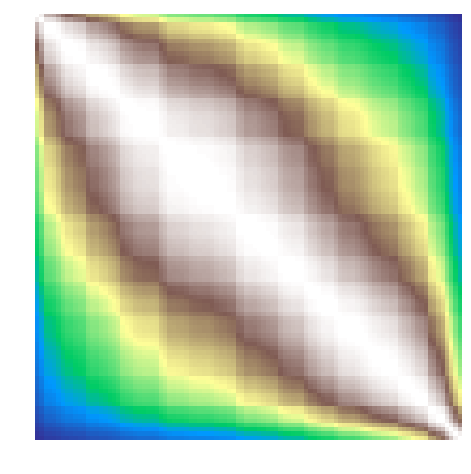In :
s2 = 11
#K(x*,x*)
K_ss = np.asmatrix(kernel.K(grid,grid))
#K(x*,x)
K_xs = np.asmatrix(kernel.K(X[:, None],grid))

mean_f_fitted  = K_xs.T*np.linalg.inv(K_xx+s2*np.eye(100))*Y[:,None]
cov_f_fitted = K_ss + s2- K_xs.T*np.linalg.inv(K_xx+s2*np.eye(100))*K_xs+s2

In :
rv_posterior_fitted = sp.random.multivariate_normal(size = 200,
mean = np.asarray(mean_f_fitted)[:,0],
cov = cov_f_fitted)
plt.plot(X,Y,"bo")
for draw in rv_posterior_fitted:
plt.plot(grid, draw, "r-", alpha=.1);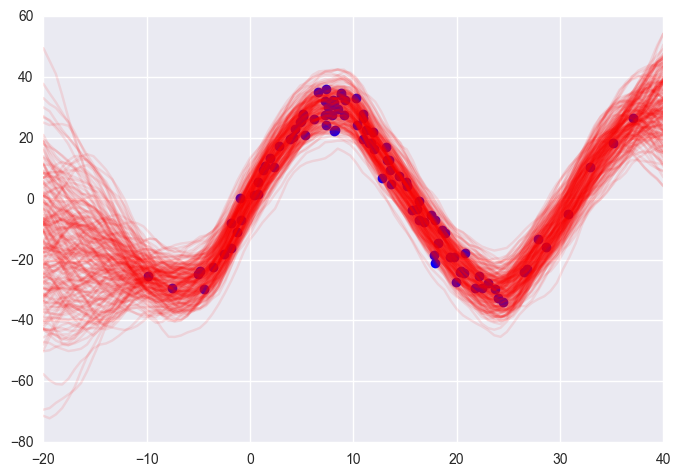Of course, GPy has built in plotting:

In :
m.plot();

 /Users/fonnescj/anaconda3/envs/bios8366/lib/python3.5/site-packages/matplotlib/figure.py:1742: UserWarning:This figure includes Axes that are not compatible with tight_layout, so its results might be incorrect.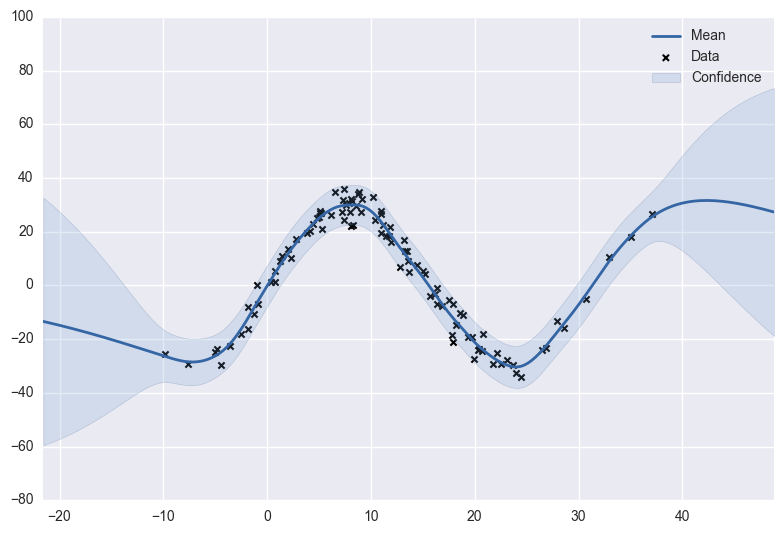### Exercise: Covariance functions¶

Perform the regression using a different covariance function. The library of kernels for GPy are found in GPy.kern

In :
# Write answer here


## Gaussian Processes in PyMC¶

GPs can be coded by hand in PyMC3:

In :
from pymc3 import Model, MvNormal, HalfCauchy, sample, traceplot, summary, Metropolis, NUTS, Deterministic
import theano.tensor as T
from theano import shared
from theano.tensor.nlinalg import matrix_inverse

In :
x = np.array([-5, -4.9, -4.8, -4.7, -4.6, -4.5, -4.4, -4.3, -4.2, -4.1, -4,
-3.9, -3.8, -3.7, -3.6, -3.5, -3.4, -3.3, -3.2, -3.1, -3, -2.9,
-2.8, -2.7, -2.6, -2.5, -2.4, -2.3, -2.2, -2.1, -2, -1.9, -1.8,
-1.7, -1.6, -1.5, -1.4, -1.3, -1.2, -1.1, -1, -0.9, -0.8, -0.7,
-0.6, -0.5, -0.4, -0.3, -0.2, -0.1, 0, 0.1, 0.2, 0.3, 0.4, 0.5,
0.6, 0.7, 0.8, 0.9, 1, 1.1, 1.2, 1.3, 1.4, 1.5, 1.6, 1.7, 1.8,
1.9, 2, 2.1, 2.2, 2.3, 2.4, 2.5, 2.6, 2.7, 2.8, 2.9, 3, 3.1,
3.2, 3.3, 3.4, 3.5, 3.6, 3.7, 3.8, 3.9, 4, 4.1, 4.2, 4.3, 4.4,
4.5, 4.6, 4.7, 4.8, 4.9, 5])

y = np.array([1.04442478194401, 0.948306088493654, 0.357037759697332, 0.492336514646604,
0.520651364364746, 0.112629866592809, 0.470995468454158, -0.168442254267804,
0.0720344402575861, -0.188108980535916, -0.0160163306512027,
-0.0388792158617705, -0.0600673630622568, 0.113568725264636,
0.447160403837629, 0.664421188556779, -0.139510743820276, 0.458823971660986,
0.141214654640904, -0.286957663528091, -0.466537724021695, -0.308185884317105,
-1.57664872694079, -1.44463024170082, -1.51206214603847, -1.49393593601901,
-2.02292464164487, -1.57047488853653, -1.22973445533419, -1.51502367058357,
-1.41493587255224, -1.10140254663611, -0.591866485375275, -1.08781838696462,
-0.800375653733931, -1.00764767602679, -0.0471028950122742, -0.536820626879737,
-0.151688056391446, -0.176771681318393, -0.240094952335518, -1.16827876746502,
-0.493597351974992, -0.831683011472805, -0.152347043914137, 0.0190364158178343,
-1.09355955218051, -0.328157917911376, -0.585575679802941, -0.472837120425201,
-0.503633622750049, -0.0124446353828312, -0.465529814250314,
-0.101621725887347, -0.26988462590405, 0.398726664193302, 0.113805181040188,
0.331353802465398, 0.383592361618461, 0.431647298655434, 0.580036473774238,
0.830404669466897, 1.17919105883462, 0.871037583886711, 1.12290553424174,
0.752564860804382, 0.76897960270623, 1.14738839410786, 0.773151715269892,
0.700611498974798, 0.0412951045437818, 0.303526087747629, -0.139399513324585,
-0.862987735433697, -1.23399179134008, -1.58924289116396, -1.35105117911049,
-0.990144529089174, -1.91175364127672, -1.31836236129543, -1.65955735224704,
-1.83516148300526, -2.03817062501248, -1.66764011409214, -0.552154350554687,
-0.547807883952654, -0.905389222477036, -0.737156477425302, -0.40211249920415,
0.129669958952991, 0.271142753510592, 0.176311762529962, 0.283580281859344,
0.635808289696458, 1.69976647982837, 1.10748978734239, 0.365412229181044,
0.788821368082444, 0.879731888124867, 1.02180766619069, 0.551526067300283])

N = len(y)


We need to code our kernel, in this case, an exponential kernel.

In :
squared_distance = lambda x, y: np.array([[(x[i] - y[j])**2 for i in range(len(x))] for j in range(len(y))])


We place Half Cauchy priors on the hyperparameters

In :
with Model() as gp_fit:

μ = np.zeros(N)

η_sq = HalfCauchy('η_sq', 5)
ρ_sq = HalfCauchy('ρ_sq', 5)
σ_sq = HalfCauchy('σ_sq', 5)

D = squared_distance(x, x)

# Squared exponential
Σ = T.fill_diagonal(η_sq * T.exp(-ρ_sq * D), η_sq + σ_sq)

obs = MvNormal('obs', μ, cov=Σ, observed=y)

Applied log-transform to η_sq and added transformed η_sq_log_ to model.
Applied log-transform to ρ_sq and added transformed ρ_sq_log_ to model.
Applied log-transform to σ_sq and added transformed σ_sq_log_ to model.

In :
with gp_fit:

# Prediction over grid
xgrid = np.linspace(-6, 6)
D_pred = squared_distance(xgrid, xgrid)
D_off_diag = squared_distance(x, xgrid)

# Covariance matrices for prediction
Σ_pred = η_sq * T.exp(-ρ_sq * D_pred)
Σ_off_diag = η_sq * T.exp(-ρ_sq * D_off_diag)

# Posterior mean
μ_post = Deterministic('μ_post', T.dot(T.dot(Σ_off_diag, matrix_inverse(Σ)), y))
# Posterior covariance
Σ_post = Deterministic('Σ_post', Σ_pred - T.dot(T.dot(Σ_off_diag, matrix_inverse(Σ)), Σ_off_diag.T))

In :
with gp_fit:
gp_trace = sample(1000)

Assigned NUTS to η_sq_log_
Assigned NUTS to ρ_sq_log_
Assigned NUTS to σ_sq_log_
100%|██████████| 1000/1000 [00:31<00:00, 31.99it/s]

In :
traceplot(gp_trace, varnames=['η_sq', 'ρ_sq', 'σ_sq']);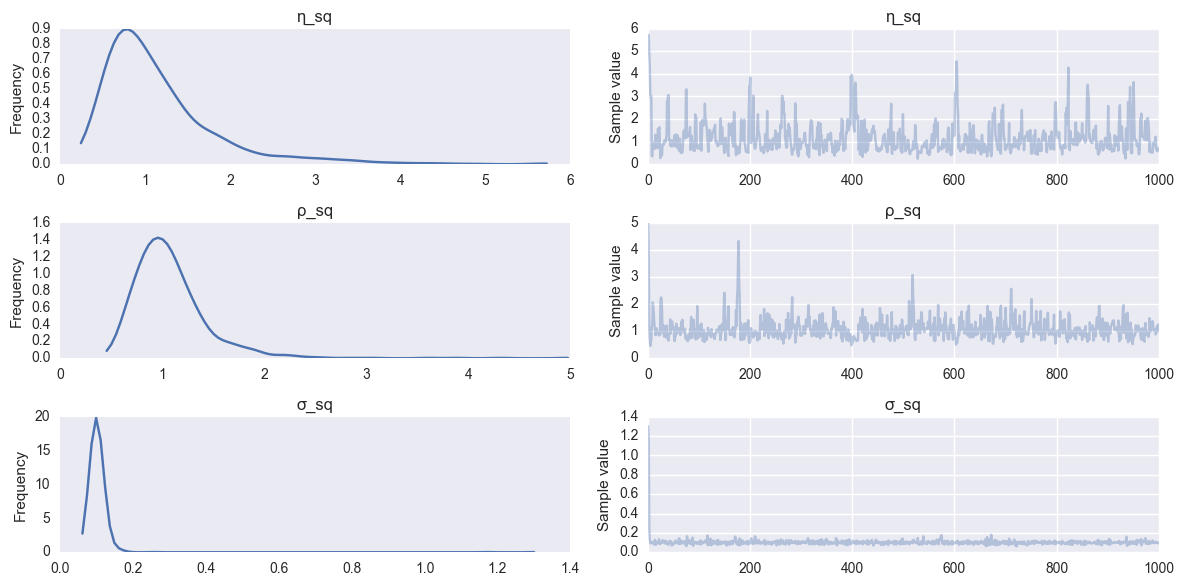In :
y_pred = [np.random.multivariate_normal(m, S) for m,S in zip(gp_trace['μ_post'][-100:], gp_trace['Σ_post'][-100:])]

 /Users/fonnescj/anaconda3/envs/bios8366/lib/python3.5/site-packages/ipykernel/__main__.py:1: RuntimeWarning:covariance is not positive-semidefinite.

In :
plt.plot(x, y, 'ro')

for yp in y_pred:
plt.plot(np.linspace(-6, 6), yp, 'b-', alpha=0.1);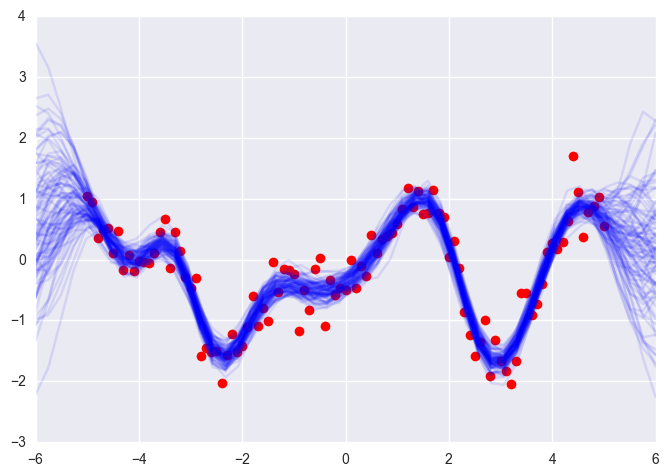## Example: Nashville daily temperatures¶

The file TNNASHVI.txt in your data directory contains daily temperature readings for Nashville, courtesy of the Average Daily Temperature Archive. This data, as one would expect, oscillates annually. Use a Gaussian process to fit a regression model to this data.

In :
import pandas as pd

daily_temps = pd.read_table("../data/TNNASHVI.txt", sep='\s+',
names=['month','day','year','temp'], na_values=-99)

In :
temps_2010 = daily_temps.temp[daily_temps.year>2010]
temps_2010.plot(style='b.', figsize=(10,6), grid=False)

Out:
<matplotlib.axes._subplots.AxesSubplot at 0x1231e56d8>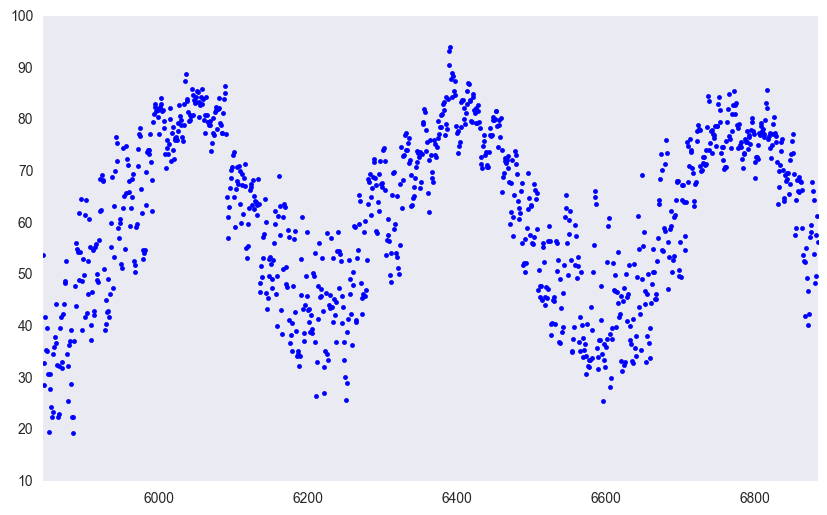In [ ]:
# Write your answer here